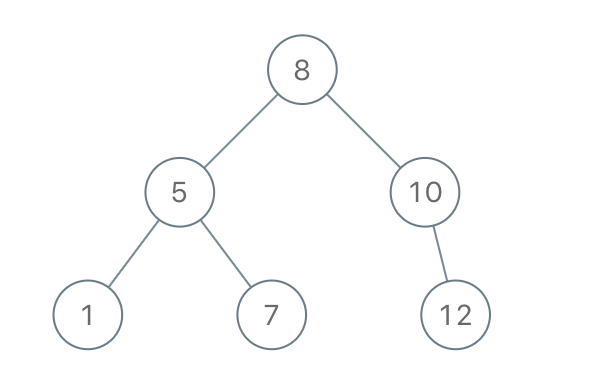# GeetCode Hub

Given an array of integers preorder, which represents the preorder traversal of a BST (i.e., binary search tree), construct the tree and return its root.

It is guaranteed that there is always possible to find a binary search tree with the given requirements for the given test cases.

A binary search tree is a binary tree where for every node, any descendant of `Node.left` has a value strictly less than `Node.val`, and any descendant of `Node.right` has a value strictly greater than `Node.val`.

A preorder traversal of a binary tree displays the value of the node first, then traverses `Node.left`, then traverses `Node.right`.

Example 1:```Input: preorder = [8,5,1,7,10,12]
Output: [8,5,10,1,7,null,12]
```

Example 2:

```Input: preorder = [1,3]
Output: [1,null,3]
```

Constraints:

• `1 <= preorder.length <= 100`
• `1 <= preorder[i] <= 108`
• All the values of `preorder` are unique.

/** * Definition for a binary tree node. * public class TreeNode { * int val; * TreeNode left; * TreeNode right; * TreeNode() {} * TreeNode(int val) { this.val = val; } * TreeNode(int val, TreeNode left, TreeNode right) { * this.val = val; * this.left = left; * this.right = right; * } * } */ class Solution { public TreeNode bstFromPreorder(int[] preorder) { } }